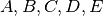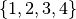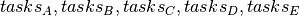The problem consists in finding a sequence of starting times of various activities, namely, of a given plant. Each activity got 5 different time slots for its starting time, represented by the set. Due to some plant requirements, two-by-two constraints exists upon the starting time activities :

• B can’t begin at 3 ;

• C can’t begin at 2 ;

• A and B can’t begin at the same starting time ;

• B and C can’t begin at the same starting time ;

• C must begin after D ;

• E must begin after A ;

• E must begin after B ;

• E must begin after C ;

• E must begin after D ;

• B and D can’t begin at the same starting time ;

• A and D must begin at the same time.

## Model formulation¶

To represent the activities starting time we defined as many variables as activities, with a domain of integer from. Constraints can be expressed as implicit constraints but also through a global constraint : a generic arc-consistency (GAC) constraint. It mainly proceeds by examining the arcs between tuples of variables and removes those values from its domain which aren’t consistent with the constraint.

The implicit constraints can be written as :And they can be expressed through a test function that validate or not a given starting time combination:

```bool StartingTimeCombinationOk(int a, int b, int c, int d, int e) {
return (b!=3) && (c!=2) && (a!=b) && (b!=c) && (c<d) && (a=d) && (e<a) && (e<b) && (e<c) && (e<d) && (b!=d);
}
```

The GAC constraint can be implemented through both classes `KGeneralizedArcConsistencyConstraint` and `KGeneralizedArcConsistencyTableConstraint`. Difference relies on the way the test function is used in the implementation of the constraint, and therefore the propagation algorithm behind.

## Implementation of the GAC constraint¶

In the standard version of the GAC constraint, end-user needs to create a derived class of `KGeneralizedArcConsistencyConstraint` which mainly overloads the `testIfSatisfied()` (constructor and copy-constructor are also needed). The former method is used by the propagation engine to test all valid combinations defined by the domain of variables. `testIfSatisfied()` is called each time one tuple needs to be validated.

```class StartingTimeCombination : public KGeneralizedArcConsistencyConstraint {
public:
KIntVarArray _vars;

StartingTimeCombination(KIntVarArray& vars)
: KGeneralizedArcConsistencyConstraint(vars,GENERALIZED_ARC_CONSISTENCY, "StartingTimeCombinationGAC"),_vars(vars)
{}

~StartingTimeCombination() {
}

bool testIfSatisfied(int * vals)
{
int _a = vals;int _b = vals;int _c = vals;int _d = vals;int _e = vals;

return StartingTimeCombinationOk(_a, _b, _c, _d, _e);
}

StartingTimeCombination(const StartingTimeCombination& toCopy) : KGeneralizedArcConsistencyConstraint(toCopy)
{
_vars = KIntVarArray(toCopy._vars);
}
};
```

Finally the complete task assignment example implementation is :

```class StartingTimeCombination : public KGeneralizedArcConsistencyConstraint {
public:
KIntVarArray _vars;

StartingTimeCombination(KIntVarArray& vars)
: KGeneralizedArcConsistencyConstraint(vars,GENERALIZED_ARC_CONSISTENCY, "StartingTimeCombinationGAC"),_vars(vars)
{}

~StartingTimeCombination() {
}

bool testIfSatisfied(int * vals)
{
int _a = vals;int _b = vals;int _c = vals;int _d = vals;int _e = vals;

return StartingTimeCombinationOk(_a, _b, _c, _d, _e);
}

StartingTimeCombination(const StartingTimeCombination& toCopy) : KGeneralizedArcConsistencyConstraint(toCopy)
{
_vars = KIntVarArray(toCopy._vars);
}

};

/////////////////////////////////////////////
/////////////////////////////////////////////
const char * TaskName[] = {"A","B","C","D","E"};
int N=5;

/////////////////////////////////////////////
// Create the task activities variables
/////////////////////////////////////////////
char name;
}

///////////////////////////////////////////////
///////////////////////////////////////////////

problem.post(gacConstraint);

/////////////////////////////////////////////
// Solve the problem
// creation of the solver
/////////////////////////////////////////////
KSolver solver(problem);

/////////////////////////////////////////////
// propagating problem
/////////////////////////////////////////////
if (problem.propagate()) {
printf("Problem is infeasible\n");
return 1;
}

solver.solve();

/////////////////////////////////////////////
// solution printing
/////////////////////////////////////////////
KSolution * sol = &problem.getSolution();

/////////////////////////////////////////////
// print solution resume
/////////////////////////////////////////////
sol->printResume();

cout << "### Computation time = " << solver.getDblAttrib(KSolver::ComputationTime) << endl;
cout << "### Number of nodes = " << solver.getIntAttrib(KSolver::NumberOfNodes) << endl;
cout << "### Depth = " << solver.getIntAttrib(KSolver::Depth) << endl;

return 0;
}
```

## Implementation of the GAC Table constraint¶

In the following GAC constraint (`KGeneralizedArcConsistencyTableConstraint` version), end-user needs this time to enumerate the complete list of valid tuples that defined the valid support of the variables with respect to the constraint. This list of tuple will be used by the propagation engine to filter the domain variables, as it is done with `testIfSatisfied()` in `KGeneralizedArcConsistencyConstraint`. This implementation is identified as the one to be preferred when the list of valid tuples is greatly inferior to the overall combinatorial of the variables.

The implementation is:

```/////////////////////////////////////////////
/////////////////////////////////////////////
const char * TaskName[] = {"A","B","C","D","E"};
int N=5;

/////////////////////////////////////////////
// Create the task activities variables
/////////////////////////////////////////////
char name;
}

///////////////////////////////////////////////
///////////////////////////////////////////////
KTupleArray tuple;
int _a, _b, _c, _d, _e;
for (_a = 0; _a <= 4; _a++) for (_b = 0; _b <= 4; _b++) for (_c = 0; _c <= 4; _c++) for (_d = 0; _d <= 4; _d++) for (_e = 0; _e <= 4; _e++) {
if (StartingTimeCombinationOk(_a, _b, _c, _d, _e)) {
KIntArray tuple_elem(N);
tuple_elem[A] = _a; tuple_elem[B] = _b; tuple_elem[C] = _c; tuple_elem[D] = _d; tuple_elem[E] = _e;
}
}

problem.post(gacTableConstraint);

/////////////////////////////////////////////
// Solve the problem
// creation of the solver
/////////////////////////////////////////////
KSolver solver(problem);

/////////////////////////////////////////////
// propagating problem
/////////////////////////////////////////////
if (problem.propagate()) {
printf("Problem is infeasible\n");
return 1;
}

solver.solve();

/////////////////////////////////////////////
// solution printing
/////////////////////////////////////////////
KSolution * sol = &problem.getSolution();

/////////////////////////////////////////////
// print solution resume
/////////////////////////////////////////////
sol->printResume();

cout << "### Computation time = " << solver.getDblAttrib(KSolver::ComputationTime) << endl;
cout << "### Number of nodes = " << solver.getIntAttrib(KSolver::NumberOfNodes) << endl;
cout << "### Depth = " << solver.getIntAttrib(KSolver::Depth) << endl;

return 0;
```